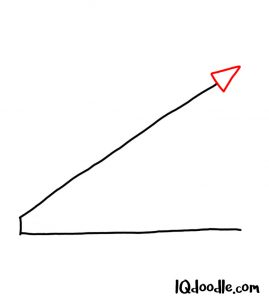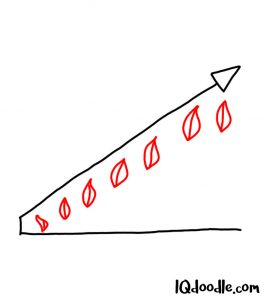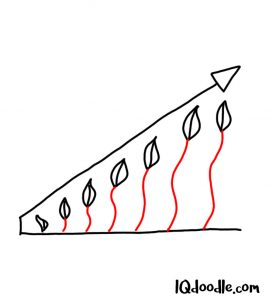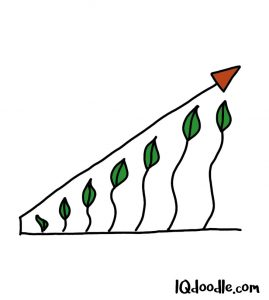## How to Doodle Growth

### Step 1If someone asked you to visualize the idea of GROWTH or of something GROWING, what would you draw? How about a graph of growing plants. Here is what it would look like. First, let’s draw the outside of the graph. Use three straight lines as shown within the example. Notice the angles of the lines, especially the top long line that’s pointing upwards on a 45-degree angle.

### Step 2To show that there is an upward movement here, let’s turn this line into an arrow. To do this, draw a triangle at the end of this line. Notice how the triangle is pointing upward at a 45-degree angle.

### Step 3Let’s now draw a graph that shows a progressive increase over time. One of the best and simplest ways to visualize growth is by drawing plants. So, for this example, let’s doodle leaves or a closed flower bud. Notice how within the example these flower buds have been draw progressively higher. Notice also how the height of the flower buds mirrors the angle of our graph line.

### Step 4Let’s now draw the stem of our flower. To do this, use curvy lines as shown within the example. Notice how the lines get progressively longer as we move from left to right along the graph.

### Step 5Add the finishing touches to your GROWTH doodle by giving it some color. And there you have it. This is our representation of GROWTH. You can, of course, substitute the flower buds or leaves with another item. This is just one example. And there are many more ways we could potentially visualize the idea of GROWTH. What other ways can you think of?